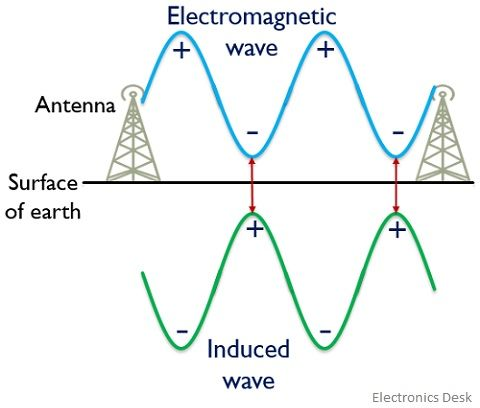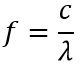# Ground Wave Propagation

Definition: A type of radio wave propagation that allows propagation of radio waves in the region between earth’s surface and ionosphere is known as Ground Wave Propagation. More simply we can say, in this type of propagation, the electromagnetic waves propagate near the surface of the earth. It is also known as surface wave propagation.

Ground wave propagation is generally a low-frequency signal propagation technique that shows suitability with the small range of operation in relation to distance. The operating frequency range in the case of ground wave propagation usually lies in the range from kHz to a few MHz (generally up to 2 MHz).

Here in this article, we will understand how waves are propagated from an end to another using ground as a medium.

## Content: Ground Wave Propagation

### What are electromagnetic waves?

Electromagnetic waves are generated due to the vibrations in the electric and magnetic fields. Basically, electromagnetic waves are the type of waves that hold the ability to propagate without the need for any specific medium for propagation as they propagate in free space. Radio waves, UV rays, X-rays and microwaves, etc. are examples of electromagnetic waves that are different from each other because of the operating frequency range.

These waves hold the ability to propagate with the speed of light (i.e., 3*108 m/s). The basic feature possessed by an electromagnetic wave is that the electric and magnetic fields are mutually perpendicular to the direction of propagation.

The figure below shows the wavelength distribution of various electromagnetic waves:We know that radio waves hold the least frequency range among the electromagnetic waves and thus have the highest wavelength. Similarly, gamma rays are high-frequency range electromagnetic waves thus have the lowest wavelength among electromagnetic waves.

### What is wave propagation?

We have already discussed the basics of the antenna along with its mechanism of operation in our previous article.

Wave propagation defines the way in which waves are transmitted from an end to another. Basically, electromagnetic waves are allowed to be propagated from transmitting antenna to receiving through a channel of free space. So, the transmission of radio waves in space from an end to another can be done using any one of the three ways:

## What is Ground Wave Propagation?

The name itself is indicating that in the ground wave propagation, the electromagnetic waves are propagated from an end to the other through the surface of the ground by gliding over it.

We know the propagation of electromagnetic waves comes under wireless communication in which the space between transmitting and receiving antenna is utilized for the propagation of the signal. So, for this, the two ends must necessarily contain transmitting and receiving antenna in order to allow signal propagation.

The electromagnetic wave from transmitting antenna propagates and gets collected by the receiving antenna. Therefore, we can say that for the propagation of electromagnetic waves, the last element of the transmitting end must be a transmitting antenna and the first element of receiving end must be a receiving antenna. So, in the ground wave propagation, the two antennas (i.e., transmitting and receiving) placed in the ground allow propagation of the signal in the form of electromagnetic waves through the earth’s surface.

Let us now understand how ground wave propagation takes place. Consider the figure given here:In the above figure, it is clearly shown that two antennas are placed on the surface of the earth. We have already discussed in the beginning that ground wave propagation allows short-distance operation. Thus the transmitting and receiving antennas must not be present at a very large distance with respect to each other.

Basically when the signal is transmitted or received to or from the antenna then the orientation of the waves depends upon the orientation of the antenna on the ground. In the ground wave propagation, the antennas are placed vertically to the ground therefore the orientation of the waves is also vertical to the ground.

The waves when emitted from the transmitting antenna, oscillates parallel to the surface of the earth. During transmission, when wave oscillates on the surface of earth then oscillations induce a wave of equal magnitude but opposite polarity on the surface.

This is represented in the figure shown here:As we can see that the induced wave is opposite in phase with the actually transmitted electromagnetic wave. So, this causes neutralization of charges that resultantly cause a reduction in the magnitude of the field with distance.

Now the question arises, do all the opposite charges neutralize each other? So, the answer is No. The charges of opposite polarity that are placed closer to each other neutralize each other while the charges separated at a large distance do not get neutralized thus propagates along the surface. In this way, an attenuated signal is collected by the receiving antenna.

In other words, the same principle can be said in a way that the number of charges varies according to the intensity and location of the field and the flow of charges generates a current. When current flows through the surface of the earth it behaves as a leaky capacitor. And so while propagating, some amount of energy gets absorbed that causes the weakening of the transmitted wave. The energy absorbed by the surface of the earth leads to cause loss of power which is due to the resistance offered by the surface to the flow of charges.

And as a part of the signal is getting attenuated while transmission, thus higher the distance greater will be the attenuation. Therefore, ground wave propagation is suitable for only a short range of propagation distance.

• Another considerable point to be kept in mind in the case of ground wave propagation is that it is suitable for the low-frequency range of operation, usually from a few kHz to 2 MHz.

Now the question arises that why we are using ground wave propagation for only low frequencies and not for the higher ones. So the reason for this is to have the least attenuation because the high-frequency signal attenuates faster. Let us understand this:

We know that electromagnetic wave propagates with the speed of light in free space. Also,As we can see that frequency and wavelength are in an inverse relationship with each other. Thus higher the frequency lesser will be the wavelength and vice-versa.

We are also aware of the fact that bending angle, θ is directly proportional to wavelength, λ. Thus higher the wavelength, the greater will be the bending, resultantly causing less amount of distortion in the presence of an obstacle. As low-frequency waves will possess a higher bending angle thus lower attenuation. But the attenuation will be higher when the frequency is high because in this case, the wavelength is small. So, we can say that waves of greater wavelength bend more efficiently hence ground wave propagation is suitable for low-frequency operation.

### Advantages of Ground Wave Propagation

1. The factor of large wavelength supports efficient bending thereby causing less attenuation.
2. The signal loss due to atmospheric conditions is less.

### Disadvantages of Ground Wave Propagation

1. The distance between the transmitting and receiving end must not be very large.
2. The operational frequency range is limited to up to 2MHz.

### Applications

Ground wave propagation finds its applications in various fields like television signal broadcasting, target detection for military purposes, radio signal transmission, and in all such applications that require a distance of operation in the local range.